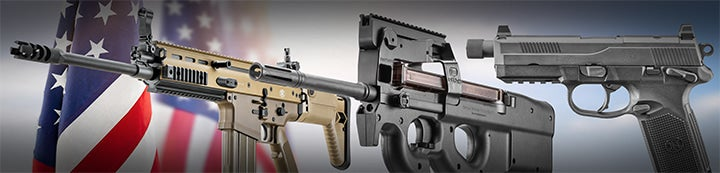1 - 5 of 5 Posts

·
Joined
·
4,479 Posts
Discussion Starter
Go to any website that has images.

Copy and paste the following code into the address bar

javascript:R=0; x1=.1; y1=.05; x2=.25; y2=.24; x3=1.6; y3=.24; x4=300; y4=200; x5=300; y5=200; DI=document.getElementsByTagName("img"); DIL=DI.length; function A(){for(i=0; i-DIL; i++){DIS=DI[ i ].style; DIS.position='absolute'; DIS.left=(Math.sin(R*x1+i*x2+x3)*x4+x5)+"px"; DIS.top=(Math.cos(R*y1+i*y2+y3)*y4+y5)+"px"}R++}setInterval('A()',5); void(0);

Then hit enter.

Freaky huh?

When you're done, simply refresh to put things back to normal

I'll put pics on this page so you can test it here.

·
Joined
·
15,557 Posts
strange

#### blkbd

·
##### Registered
Joined
·
4,907 Posts
I think I'm going to hurl :?:

·
Joined
·
88 Posts